# 7.3: Second-order differential equations

•• Contributed by Peter Woolf et al.
• Assistant Professor (Chemical Engineering) at University of Michigan

## 3.1 Introduction

We consider the general Second-order differential equation:

$\tau^{2} \frac{d^{2} Y(t)}{d t^{2}}+2 \zeta \tau \frac{d Y(t)}{d t}+Y(t)=X(t)$

If you expand the previous Second-order differential equation:

$\tau_{1} \tau_{2} \frac{d^{2} Y(t)}{d t^{2}}+\left(\tau_{1}+\tau_{2}\right) \frac{d Y(t)}{d t}+Y(t)=X(t)$

$\left(\tau_{1} \frac{d}{d t}+1\right)\left(\tau_{2} \frac{d}{d t}+1\right) Y(t)=X(t)$

where:

$\tau=\sqrt{\tau_{1} \tau_{2}}$

$\zeta=\frac{\tau_{1}+\tau_{2}}{2 \sqrt{\tau_{1} \tau_{2}}}$

Expansion of the differential equation allows you to guess what the shape of the solution (Y(t)) will look like when X(t)=1.

The following rules apply when τ1 = Re(τ1)+ i*Im(τ1) and τ2 = Re(τ2)+ i*Im(τ2):

• If Re(τ1) and Re(τ2) are both positive, and there are NO imaginary parts, Y(t) will exponentially decay (overdamped).
• If Re(τ1) and Re(τ2) are both positive, and there ARE imaginary parts, Y(t) will oscillate until it reaches steady state (underdamped).
• If Re(τ1) and Re(τ2) are both negative, and there are NO imaginary parts, Y(t) will exponentially grow (unstable).
• If Re(τ1) and Re(τ2) are both negative, and there ARE imaginary parts, Y(t) will oscillate and grow exponentially (unstable).
• If Re(τ1) and Re(τ2) are both zero, and there ARE imaginary parts, Y(t) will oscillate and neither grow nor decay.
• If τ1 and τ2 are both zero, Y(t) is equal to X(t).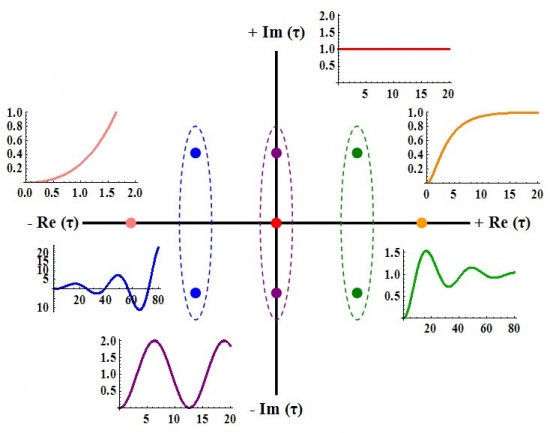## 3.2 Solution of the General Second-Order System (When X(t)= θ(t))

The solution for the output of the system, Y(t), can be found in the following section, if we assume that the input, X(t), is a step function θ(t). The solution will depend on the value of ζ. If ζ is less than one, Y(t) will be underdamped. This means that the output will overshoot and oscillate. If ζ is equal to one, Y(t) will be critically damped. This means that the output will reach the steady state value quickly, without overshoot or oscillation. If ζ is greater than one, Y(t) will be overdamped. This means that the output will not reach the steady state value as quickly as a critically damped system, but there will be no overshoot or oscillation.

### 3.2.1 Underdamped (ζ<1)

If ζ < 1, the solution is:Where:

$\phi=-\tan ^{-1}\left(\frac{\sqrt{1-\zeta^{2}}}{\zeta}\right)$The decay ratio (C/A) can be calculated using the following equation:The overshoot (A/B) can be calculated using the following equation:The period (T) and the frequency (ω) are the following:

$T=t_{2}-t_{1}=\frac{2 \pi \tau}{\sqrt{1-\zeta^{2}}}$

$\omega=\frac{2 \pi}{T}=\frac{\sqrt{1-\zeta^{2}}}{\tau}$

### 3.2.2 Critically Damped (ζ=1)

If ζ = 1, the solution is:

$Y(t)=1-\left(1+\frac{t}{\tau}\right) e^{-t / \tau}$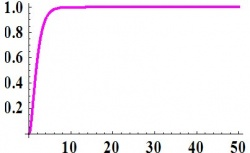### 3.2.3 Overdamped (ζ>1)

If $$ζ > 1$$, the solution is:where:

$\phi=-\tanh ^{-1}\left(\frac{\sqrt{\zeta^{2}-1}}{\zeta}\right)$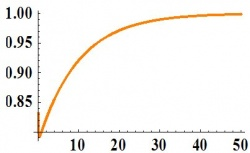## 3.3 Example

### 3.3.1 Problem:

Given:

• A1 = 1 m2
• A2 = 1.5 m2
• R1 = 0.25 s/m2
• R2 = 0.75 s/m2

where:

• A is the area of the tank
• Q is the volumetric flowrate
• R is the resistance to the flow of the stream exiting the tank
• H is the height of liquid in the tank

develop an expression describing the response of H2 to Qin. Determine if the system is over, under or critically damped and determine what the graph of the expression would look like using the complex τ plane above. A diagram of the system is shown below:### 3.3.2 Solution:

Performing a mass balance on each tank:

$A_{1} \frac{d H_{1}}{d t}=Q_{i n}-\frac{H_{1}}{R_{1}} \label{1}$

$A_{2} \frac{d H_{2}}{d t}=\frac{H_{1}}{R_{1}}-\frac{H_{2}}{R_{2}} \label{2}$

where the left hand terms account for the accumulation in the tank and the right hand terms account for the flow in the entering and exiting streams

Let τ1 = R1A1 and τ2 = R2A2

Equations (1) and (2) now become

$\tau_{1} \frac{d H_{1}}{d t}=R_{1} Q_{i n}-H_{1} \label{3}$

$\tau_{2} \frac{d H_{2}}{d t}=\frac{R_{2}}{R_{1}} H_{1}-H_{2} \label{4}$

Put like terms on the same side and factor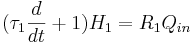(5)(6)

Apply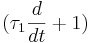operator from equation (5) to equation (6)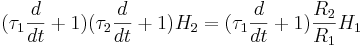(7)

Theterm from the left hand portion of equation (5) can be substituted into the right hand side of equation (7)This expression shows the response of H2 to Qin as a second order solution like those pictured above. Here Y(t)=H2 and X(t)=R2 QinBoth values of τ are positive real numbers, and the behavior of the graph of the equation can be found on the complex τ plane above.

## 3.4 Analogy to Physics: Spring System

It might be helpful to use a spring system as an analogy for our second order systems.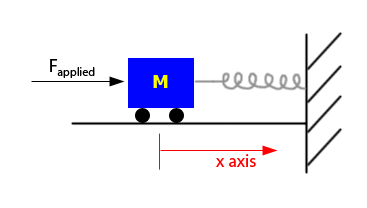From Newton's second law of motion,

F = ma

Where:

F is Force

m is mass

a is acceleration

For the spring system, this equation can be written as:

FappliedFfrictionFrestoring = mx''

Where:

x'' is the acceleration of the car in the x-direction

Fappliedfx' − kx = mx''

Where:

k is the spring constant, which relates displacement of the object to the force applied

f is the frequency of oscillationAs you can see, this equation resembles the form of a second order equation. The equation can be then thought of as:

τ2x'' + 2ζτx' + x = Fapplied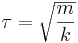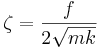Because of this, the spring exhibits behavior like second order differential equations:

If ζ > 1 or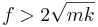it is overdamped

If ζ = 1 orit is critically damped

If ζ < 1 orit is underdamped